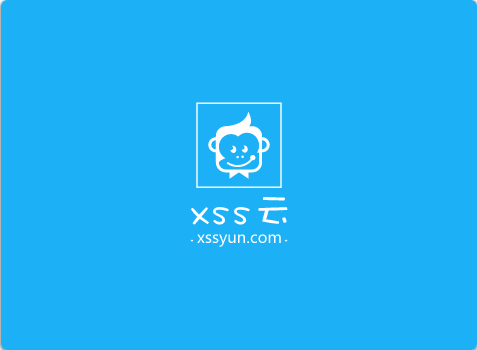# 浅谈Go类型转换之间的那些事站长

surging 社区版本支持.net core 3.1

#### 试着答一答这些问题

##### s[i]和(for _,v range)的v的区别是什么
``````var s string = "AB"
fmt.Println(reflect.TypeOf(s))
for _, v := range s {
fmt.Println(reflect.TypeOf(v))
}``````
##### a.(),和 a(b) 的区别是什么？
``````var v interface{} = 1
var s uint8 = 1

temp1 := int(s)
temp2 := v.(int)

fmt.Println(temp1,temp2)``````

#### Go的类型系统了解

##### Go的类型

Go语言是一门静态编译型语言，是一门强类型语言，Go语言中类型分为两种：命名类型(已定义类型)和未命名类型(组合类型)，我举例说一下

1. 命名类型（已定义类型）
``````uint8(byte) uint16 uint32 uint64 int int8 int16 int32(rune) int64 bool string
float32 float64 complex64 complex128``````

``````//也是命名类型
type MyBool bool ``````
1. 未命名类型 (组合类型)
``slice map chan function interface struct pointer``

##### 自定义类型和底层类型

Go允许通过type关键字定义一个类型
Go的每一个类型都一个底层类型，类型的底层类型有如下规律

1. 每一个命名类型的底层类型都是自己
2. 每一个组合类型的底层类型都是自己
3. 在一个类型的声明中，新声明的类型和原类型的底层类型是共享的

``````type MyInt int
func CustomType() {
var i MyInt = 2
var j int = 1
j = i
i = j
fmt.Println(i == j)
}``````

``````//输出MyInt int
fmt.Println(reflect.TypeOf(i), reflect.TypeOf(j))
//输出int int
fmt.Println(reflect.TypeOf(i).Kind(), reflect.TypeOf(j).Kind())``````

``````type MyM struct {
i int64
}
type MyN struct {
j int32
}
func TestStruct() {
n := MyN{j: 10}
var m MyM
m = MyM(n)
fmt.Println(n,m)
}``````
##### 如何追踪朔源一个类型的的底层类型

``````type MyInt int
type I MyInt
type Ints []int
type MyInts []MyInt
type M map[string]string
type CustomM M``````

MyInt的底层类型是int
I的底层类型时int
Ints的底层类型是[]int
MyInts的底层类型是slice
M的底层类是map
CustomM的底层类是map

.NET Core 开车记：Data Protection Key 过期问题与登录页面访问慢

``reflect.TypeOf(variable).Kind()``
##### 类型别名

``````// byte is an alias for uint8 and is equivalent to uint8 in all ways. It is
// used, by convention, to distinguish byte values from 8-bit unsigned
// integer values.
type byte = uint8

// rune is an alias for int32 and is equivalent to int32 in all ways. It is
// used, by convention, to distinguish character values from integer values.
type rune = int32``````

``````var r rune = 'c'
var b byte = 1
fmt.Println(reflect.TypeOf(r).Kind()) //int32
fmt.Println(reflect.TypeOf(b).Kind()) //uint8``````

``````//相比定义一个类型多了一个=号
type alaisInt64 = int64``````
##### 类型转换和断言

``````
//这个转换时类型不同，也不兼容，所以编译报错
s := "ab"
i := int(s)

//这个转换类型不同，但兼容，所以OK
var j int8 = 1
m := int(j)

//这个转换是失败的，系统会检测到类型不匹配，直接panic
var k interface{} = "s"
l := k.(int)
//但我们可以通过一个参数来判断,只有f为true时，才会转换成功
l,f := k.(int)
//这个转换是成功的
p,f := k.(string)``````

#### 类型转换的实践，勤加练习才能理解

##### 数字类型之间转换

128的二进制：………00000000_10000000

``````//这个转换没有任何问题，都OK
var i int8 = 123
var j int16 = int16(i)
//这个转换会丢失精度，从高位转低位
var m int64 = 128
var n int8 = int8(m) //n的结果是-128，因为int8能表达的最大值是127，最小值是-128，``````
##### 字符串，字节，数字，字符互相转换
``````var s1,s2 string = "AbcD","1234"
//转字节
bs1 := []byte(s1); bs2 := []byte(s2)

//字节数组转字符串
s11 := string(bs1); s22 := string(bs2)
//单个字节转字符串
ss := string(bs1)
fmt.Println(s11, s22, ss)

//s2转数字 ,err 表示是否能转换成功，比如s1就会转换失败
i, err := strconv.Atoi(s2)
//数字转字符串
s := strconv.Itoa(i)

//字符串转字符数组
runes := []rune(s1)

//字符数组转字符串
ss1 := string(runes)
//单个字符转字符串
ss2 := strconv.QuoteRune(runes)

//字符转字节
bss := make([]byte, 0)
bss = strconv.AppendQuoteRune(bss, runes)
fmt.Println(err, s, ss1, ss2, runes, bss, string(bss))
//除开rune和byte底层的类型的区别，在使用上，
//rune能处理一切的字符，而byte仅仅局限在ascii

//整形转字节
x := int32(68)
bytesBuffer := bytes.NewBuffer([]byte{})
binary.Write(bytesBuffer, binary.BigEndian, x)
//字节转整形
var y int32
##### 接口到具体类型的转换
``````//由接口类型转换为具体的类型
var i interface{} = 1
t, f := i.(int)
if f { //转换成功
fmt.Println(t)
} else {//转换失败
fmt.Println(reflect.TypeOf(i).Kind(), reflect.TypeOf(i))
}``````Windows/Linux：VMware虚拟机与外部通过内网IP通讯2020年在家赚取零花钱

Q Q 登 录